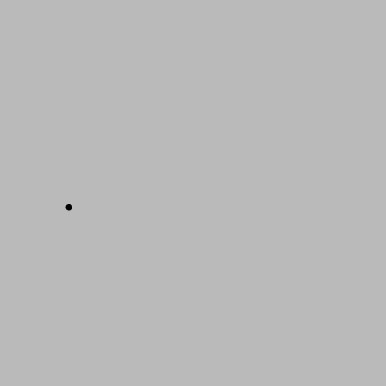# 如何能做到 101%？ABCDEFGHIJKLMNOPQRSTUVWXYZ

1 2 3 4 5 6 7 8 9 10 11 12 13 14 15 16 17 18 19 20 21 22 23 24 25 26

8+1+18+4+23+15+18+11 = 98%

11+14+15+23+12+5+4+7+5 = 96%

1+20+20+9+20+21+4+5 = 100%

L-O-V-E-O-F-G-O-D =
12+15+22+5+15+6+7+15+4 = 101%